### Symmetry - Solutions 3

CBSE Class –VII Mathematics
NCERT Solutions
Chapter 14 Symmetry (Ex. 14.3)

Question 1. Name any two figures that have both line symmetry and rotational symmetry.
Question 2. Draw, wherever possible, a rough sketch of:
1. a triangle with both line and rotational symmetries of order more than one.
2. a triangle with only line symmetry and no rotational symmetry of order more than one.
3 .a quadrilateral with a rotational symmetry of order more than one but not a line symmetry.
4. a quadrilateral with line symmetry but not a rotational symmetry of order more than one.
Answer: (i) An equilateral triangle has both line and rotational symmetries of order more than one.
Line symmetry: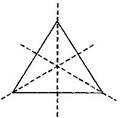Rotational symmetry: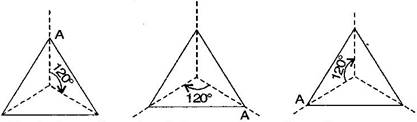(ii) An isosceles triangle has only one line of symmetry and no rotational symmetry of order more than 1.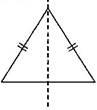Line symmetry: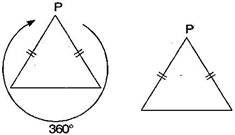Rotational symmetry:​
(iii) It is not possible because order of rotational symmetry is more than 1 of a figure, most acertain the line of symmetry.
(iv) A trapezium which has equal non-parallel sides, a quadrilateral with line symmetry but not a rotational symmetry of order more than 1.
Line symmetry: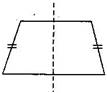Rotational symmetry: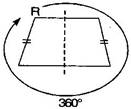Question 3. In a figure has two or more lines of symmetry, should it have rotational symmetry of order more than 1?
Answer: Yes, because every line through the centre forms a line of symmetry and it has rotational symmetry around the centre for every angle.
Question 4. Fill in the blanks:
 Shape Centre of Rotation Order of Rotation Angle of Rotation Square Rectangle Rhombus Equilateral triangle Regular hexagon Circle Semi-circle
 Shape Centre of Rotation Order of Rotation Angle of Rotation Square Intersecting point of diagonals. 4 90∘${90}^{\circ }$ Rectangle Intersecting point of diagonals. 2 180∘${180}^{\circ }$ Rhombus Intersecting point of diagonals. 2 180∘${180}^{\circ }$ Equilateral triangle Intersecting point of medians. 3 120∘${120}^{\circ }$ Regular hexagon Intersecting point of diagonals. 6 60∘${60}^{\circ }$ Circle Centre infinite At every point Semi-circle Mid-point of diameter 1 360∘${360}^{\circ }$
Question 5. Name the quadrilateral which has both line and rotational symmetry of order more than 1.
Answer: Square has both line and rotational symmetry of order more than 1.
Line symmetry: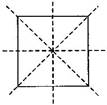Rotational symmetry: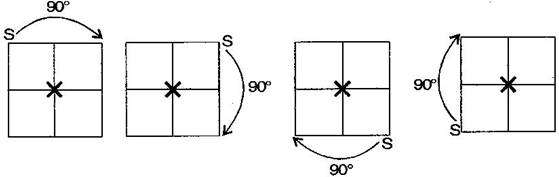Question 6. After rotating by ${60}^{\circ }$ about a centre, a figure looks exactly the same as its original position. At what other angles will this happen for the figure?
Answer: Other angles will be ${120}^{\circ },{180}^{\circ },{240}^{\circ },{300}^{\circ },{360}^{\circ }$.
For rotation:It will rotate six times.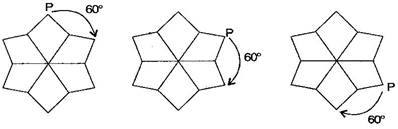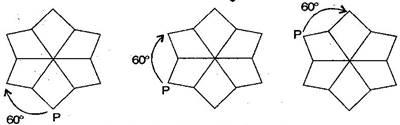For rotation:It will rotate three times.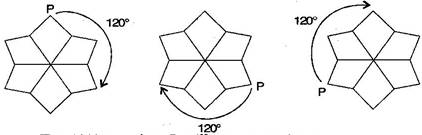For rotation:It will rotate two times.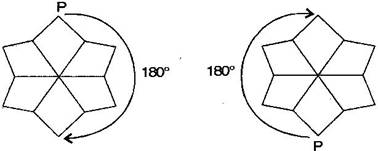For rotation:It will rotate one time.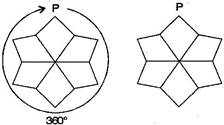Question 7. Can we have a rotational symmetry of order more than 1 whose angle of rotation is:
(i) ${45}^{\circ }$(ii) ${17}^{\circ }$ ?
Answer: (i) If the angle of rotation is ${45}^{\circ },$ then symmetry of order is possible and would be 8 rotations.
(ii) If the angle of rotational is ${17}^{\circ },$ then symmetry of order is not possible because ${360}^{\circ }$ is not complete divided by